### Affordable pricing from £10/month

In this worksheet, students complete tables for quadratic graphs.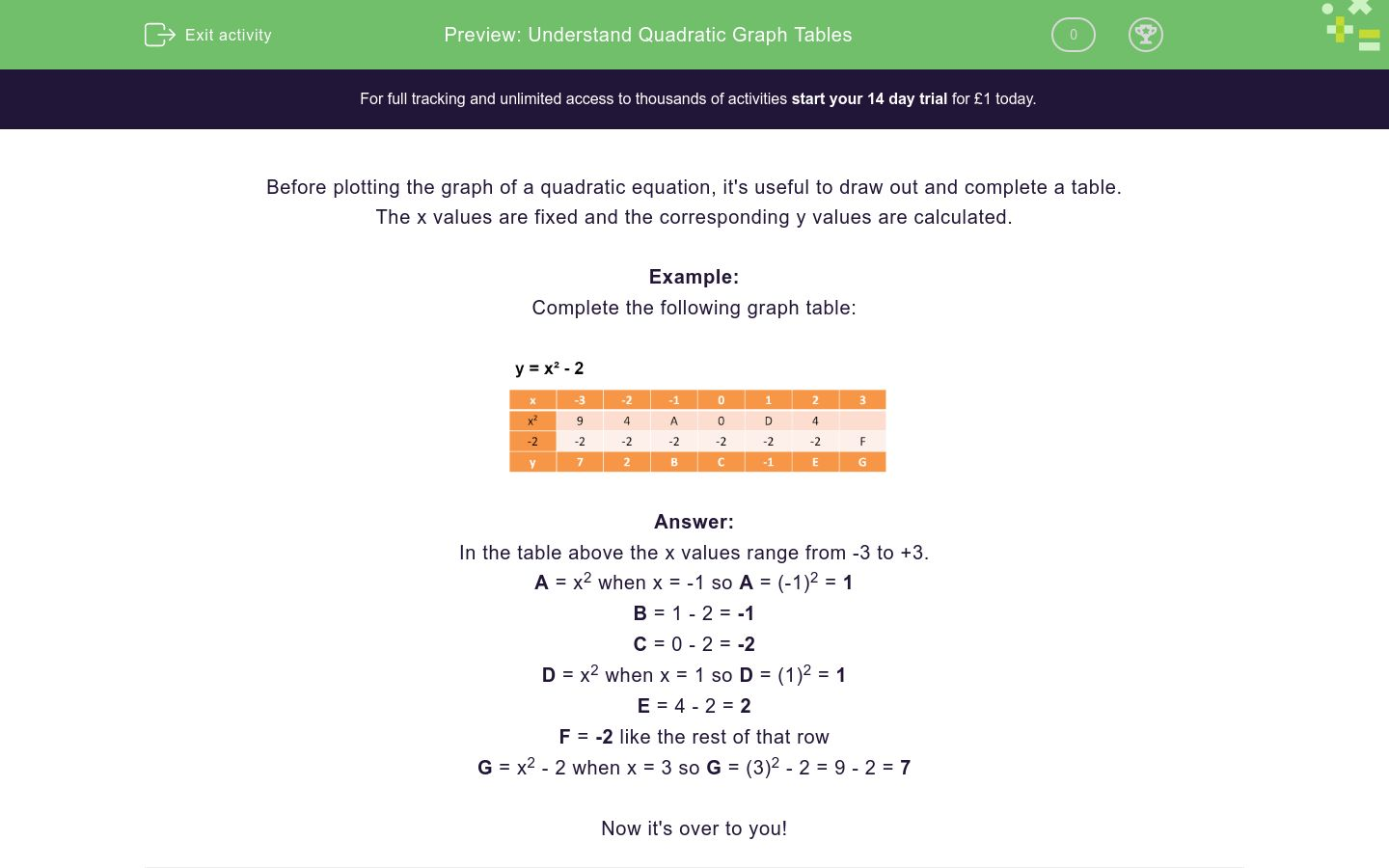Key stage:  KS 4

Curriculum topic:  Algebra

Difficulty level:### QUESTION 1 of 10

Before drawing the graph of a quadratic equation, it is helpful to draw out and complete a table.

The x values are fixed and the corresponding y values are calculated.

Example

Complete the following graph table.Here the x values range from -3 to +3.

A = x2 when x = -1 so A = (-1)2 = 1

B = 1 - 2 = -1

C = 0 - 2 = -2

D = x2 when x = 1 so D = (1)2 = 1

E = 4 - 2 = 2

F = -2 like the rest of that row

G = x2 - 2 when x = 3 so G = (3)2 - 2 = 9 - 2 = 7

Complete the following graph table by matching the correct values to the letters.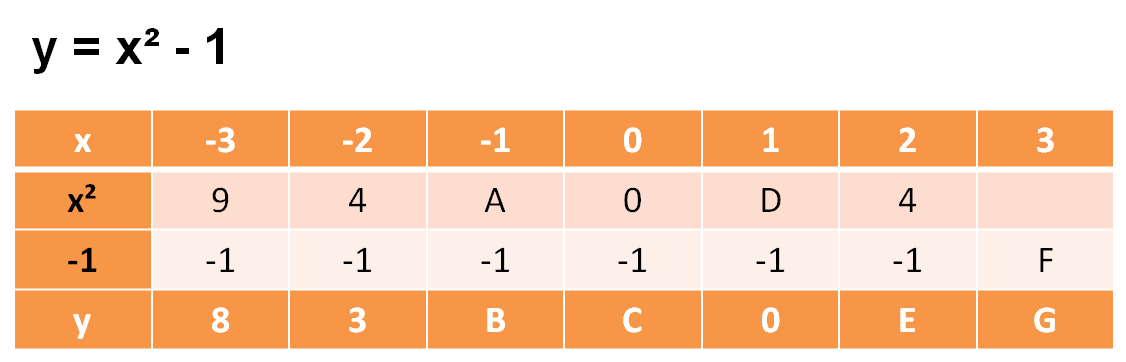## Column B

A
3
B
8
C
1
D
1
E
-1
F
-1
G
0

Complete the following graph table by matching the correct values to the letters.## Column B

A
4
B
-1
C
-5
D
-5
E
4
F
-4
G
4

Complete the following graph table by matching the correct values to the letters.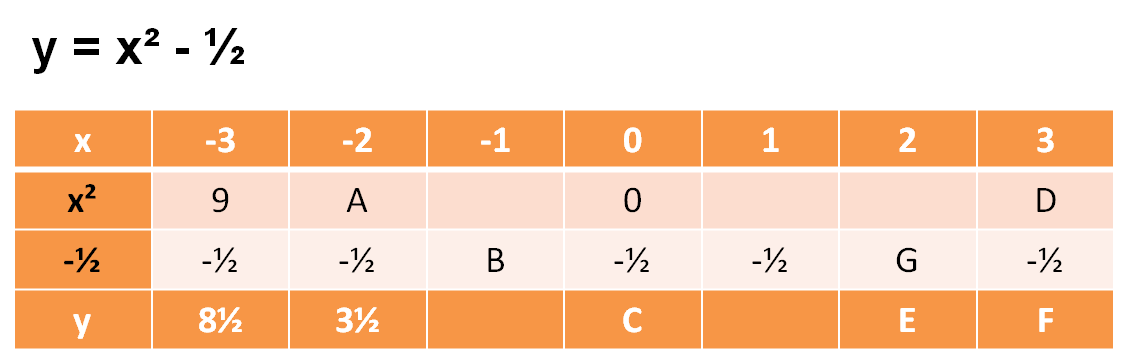## Column B

A
4
B
9
C
D
E
F
G

Complete the following graph table by matching the correct values to the letters.## Column B

A
-3
B
15
C
-3
D
4
E
18
F
5
G
8

Complete the following graph table by matching the correct values to the letters.## Column B

A
12
B
27
C
22
D
7
E
-5
F
-5
G
9

Complete the following graph table by matching the correct values to the letters.## Column B

A
36
B
2
C
66
D
146
E
2
F
64
G
144

Complete the following graph table by matching the correct values to the letters.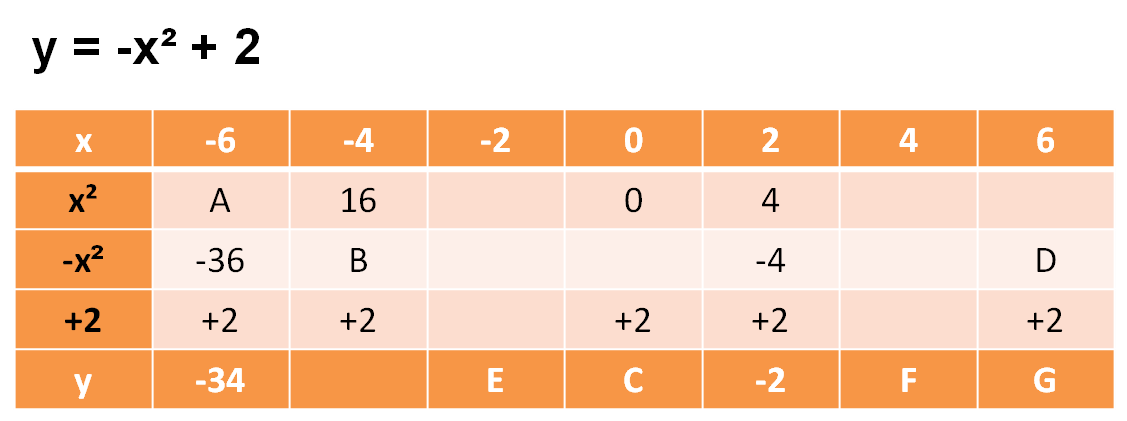## Column B

A
-2
B
-34
C
2
D
-36
E
36
F
-16
G
-14

Complete the following graph table by matching the correct values to the letters.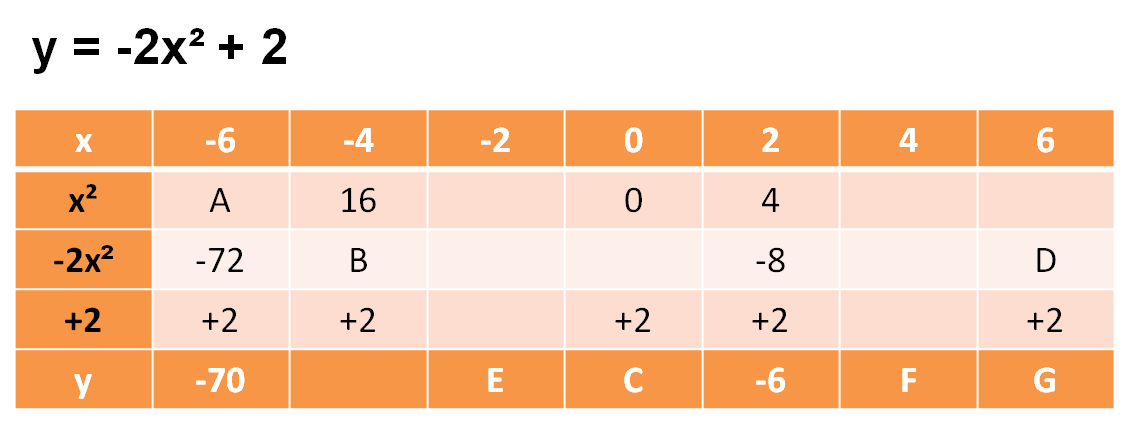## Column B

A
-6
B
2
C
-70
D
-72
E
-32
F
-30
G
36

Complete the following graph table by matching the correct values to the letters.## Column B

A
36
B
2
C
6
D
4
E
11
F
9
G
3

Complete the following graph table by matching the correct values to the letters.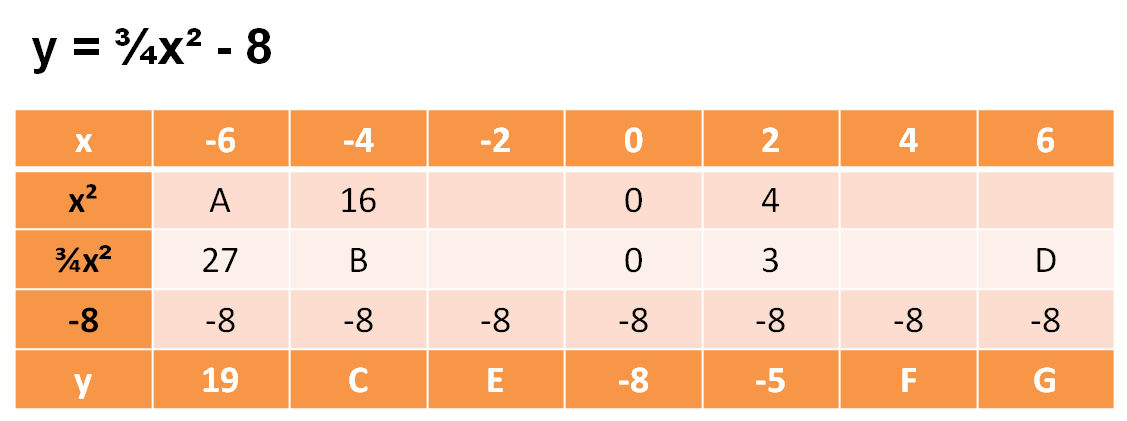## Column B

A
4
B
19
C
12
D
27
E
4
F
-5
G
36
• Question 1

Complete the following graph table by matching the correct values to the letters.## Column B

A
1
B
0
C
-1
D
1
E
3
F
-1
G
8
• Question 2

Complete the following graph table by matching the correct values to the letters.## Column B

A
4
B
-4
C
-5
D
4
E
-1
F
4
G
-5
• Question 3

Complete the following graph table by matching the correct values to the letters.## Column B

A
4
B
C
D
9
E
F
G
• Question 4

Complete the following graph table by matching the correct values to the letters.## Column B

A
4
B
8
C
-3
D
18
E
-3
F
5
G
15
• Question 5

Complete the following graph table by matching the correct values to the letters.## Column B

A
9
B
12
C
-5
D
27
E
-5
F
7
G
22
• Question 6

Complete the following graph table by matching the correct values to the letters.## Column B

A
36
B
64
C
2
D
144
E
2
F
66
G
146
• Question 7

Complete the following graph table by matching the correct values to the letters.## Column B

A
36
B
-16
C
2
D
-36
E
-2
F
-14
G
-34
• Question 8

Complete the following graph table by matching the correct values to the letters.## Column B

A
36
B
-32
C
2
D
-72
E
-6
F
-30
G
-70
• Question 9

Complete the following graph table by matching the correct values to the letters.## Column B

A
36
B
4
C
2
D
9
E
3
F
6
G
11
• Question 10

Complete the following graph table by matching the correct values to the letters.## Column B

A
36
B
12
C
4
D
27
E
-5
F
4
G
19
---- OR ----

Sign up for a £1 trial so you can track and measure your child's progress on this activity.

### What is EdPlace?

We're your National Curriculum aligned online education content provider helping each child succeed in English, maths and science from year 1 to GCSE. With an EdPlace account you’ll be able to track and measure progress, helping each child achieve their best. We build confidence and attainment by personalising each child’s learning at a level that suits them.